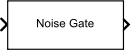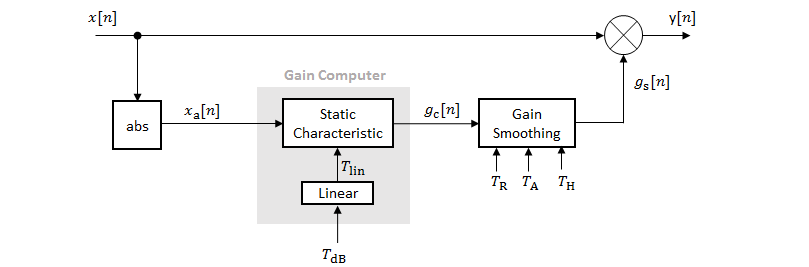# Noise Gate

Dynamic range gate

• Library:
• Audio Toolbox / Dynamic Range Control

•## Description

The Noise Gate block performs dynamic range gating independently across each input channel. Dynamic range gating suppresses signals below a given threshold. The block uses specified attack, release, and hold times to achieve a smooth applied gain curve.

## Ports

### Input

expand all

• Matrix input –– Each column of the input is treated as an independent channel.

• 1-D vector input –– The input is treated as a single channel.

This port is unnamed unless you specify additional input ports.

Data Types: `single` | `double`

#### Dependencies

To enable this port, select Specify from input port for the Threshold (dB) parameter.

Data Types: `single` | `double`

#### Dependencies

To enable this port, select Specify from input port for the Attack time (s) parameter.

Data Types: `single` | `double`

#### Dependencies

To enable this port, select Specify from input port for the Release time (s) parameter.

Data Types: `single` | `double`

#### Dependencies

To enable this port, select Specify from input port for the Hold time (s) parameter.

Data Types: `single` | `double`

### Output

expand all

The Noise Gate block outputs a signal with the same data type as the input signal. The size of the output depends on the size of the input:

• Matrix input –– The block outputs a matrix the same size and data type as the input signal.

• 1-D vector input –– The block outputs an N-by-1 matrix (column vector), where N is the number of elements in the 1-D vector.

This port is unnamed until you select the Output gain (dB) parameter.

Data Types: `single` | `double`

#### Dependencies

To enable this port, select the Output gain (dB) parameter.

Data Types: `single` | `double`

## Parameters

expand all

If a parameter is listed as tunable, then you can change its value during simulation.

Main Tab

Operation threshold is the level below which gain is applied to the input signal.

To specify Threshold (dB) from an input port, select Specify from input port for the parameter.

Tunable: Yes

The plot is updated automatically when parameters of the Noise Gate block change.

Tunable: Yes

Attack time is the time the applied gain takes to rise from 10% to 90% of its final value when the input goes below the threshold. The Attack time (s) parameter smooths the applied gain curve.

To specify Attack time (s) from an input port, select Specify from input port for the parameter.

Tunable: Yes

Release time is the time the applied gain takes to drop from 90% to 10% of its final value when the input goes above the threshold. The Release time (s) parameter smooths the applied gain curve.

To specify Release time (s) from an input port, select Specify from input port for the parameter.

Tunable: Yes

Hold time is the period for which the (negative) gain is held before starting to decrease towards its steady state value when the input level drops below the threshold.

To specify Hold time (s) from an input port, select Specify from input port for the parameter.

Tunable: Yes

When you select this parameter, the block inherits its sample rate from the input signal. When you clear this parameter, you specify the sample rate in Input sample rate (Hz).

Tunable: No

Tunable: Yes

#### Dependencies

To enable this parameter, clear the Inherit sample rate from input parameter.

When you select this parameter, an additional output port, G, is added to the block. The G port outputs the gain applied on each input channel in dB.

Tunable: No

When you select this parameter, an additional input port SC is added to the block. The SC port enables dynamic range gating of the input signal x using a separate sidechain signal.

The datatype and (frame) length input to the SC port must be the same as the input to the x port.

The number of channels of the sidechain input must be equal to the number of channels of `x` or be equal to one.

• `Sidechain channel count is equal to one` –– The computed gain, `G`, based on this channel is applied to all channels of `x`.

• ```Sidechain channel count is equal to channel count of ````x` –– The computed gain, `G`, for each sidechain channel is applied to the corresponding channel of `x`.

Tunable: No

• `Interpreted execution` –– Simulate model using the MATLAB® interpreter. This option shortens startup time and has a simulation speed comparable to ```Code generation```. In this mode, you can debug the source code of the block.

• `Code generation` –– Simulate model using generated C code. The first time you run a simulation, Simulink® generates C code for the block. The C code is reused for subsequent simulations, as long as the model does not change. This option requires additional startup time, but the speed of the subsequent simulations is comparable to ```Interpreted execution```.

Tunable: No

## Block Characteristics

 Data Types `double` | `single` Direct Feedthrough `no` Multidimensional Signals `no` Variable-Size Signals `yes` Zero-Crossing Detection `no`

## Algorithms

The Noise Gate block processes a signal frame by frame and element by element.1. The N-point signal, x[n], is converted to magnitude:

`${x}_{\text{a}}\text{[}n\right]=|x\left[n\right]|$`

2. xa[n] passes through the gain computer. The gain computer uses the static characteristic properties of the dynamic range gate to apply a brickwall gain for signal below the threshold:

`${g}_{\text{c}}\left({x}_{\text{a}}\right)=\left\{\begin{array}{cc}0& {x}_{\text{a}}<{T}_{\text{lin}}\\ 1& {x}_{\text{a}}\ge {T}_{\text{lin}}\end{array}$`

Tlin is the threshold property converted to a linear domain:

`${T}_{\text{lin}}={10}^{\left({T}_{\text{dB}}}{20}\right)}\text{\hspace{0.17em}}.$`

3. The computed gain, gc[n], is smoothed using specified attack, release, and hold time parameters:

`${g}_{\text{s}}\left[n\right]=\left\{\begin{array}{cc}{\alpha }_{\text{A}}{g}_{\text{s}}\left[n-1\right]+\left(1-{\alpha }_{\text{A}}\right){g}_{\text{c}}\left[n\right]& \text{\hspace{0.17em}}\left({C}_{\text{A}}>{T}_{\text{H}}\right)\text{\hspace{0.17em}}\text{\hspace{0.17em}}&\text{\hspace{0.17em}}\text{\hspace{0.17em}}\left({g}_{\text{c}}\left[n\right]\le {g}_{\text{s}}\left[n-1\right]\right)\\ {g}_{\text{s}}\left[n-1\right]\text{\hspace{0.17em}}& {C}_{\text{A}}\le {T}_{\text{H}}\\ {\alpha }_{\text{R}}{g}_{\text{s}}\left[n-1\right]+\left(1-{\alpha }_{\text{R}}\right){g}_{\text{c}}\left[n\right]\text{ }& {g}_{\text{c}}\left[n\right]>{g}_{\text{s}}\left[n-1\right]\end{array}$`

CA is the hold counter for attack. The limit, TH, is determined by the Hold time (s) parameter.

The attack time coefficient, αA, is calculated as

`${\alpha }_{\text{A}}=\mathrm{exp}\left(\frac{-\mathrm{log}\left(9\right)}{Fs×{T}_{\text{A}}}\right)\text{\hspace{0.17em}}.$`

The release time coefficient, αR, is calculated as

`${\alpha }_{\text{R}}=\mathrm{exp}\left(\frac{-\mathrm{log}\left(9\right)}{Fs×{T}_{\text{R}}}\right)\text{\hspace{0.17em}}.$`

TA is the attack time period, specified by the Attack time (s) parameter. T R is the release time period, specified by the Release time (s) parameter. Fs is the input sampling rate, specified by the Inherit sample rate from input or Input sample rate (Hz) parameter.

4. The output of the dynamic range gate is given as

`$y\left[n\right]=x\left[n\right]×{g}_{\text{s}}\left[n\right].$`

 Giannoulis, Dimitrios, Michael Massberg, and Joshua D. Reiss. "Digital Dynamic Range Compressor Design –– A Tutorial And Analysis." Journal of Audio Engineering Society. Vol. 60, Issue 6, 2012, pp. 399–408.

## Support평가판 신청